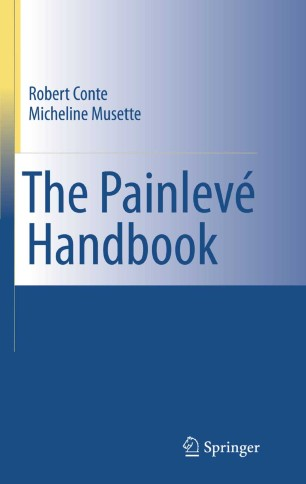# The Painlevé Handbook

• Robert Conte
• Micheline MusetteBook

1. Front Matter
Pages i-xxiii
2. Pages 1-9
3. Pages 11-31
4. Pages 33-76
5. Pages 93-144
6. Pages 145-162
7. Pages 163-186
8. Pages 187-190
9. Back Matter
Pages 191-256

### Introduction

Nonlinear differential or difference equations are encountered not only in mathematics, but also in many areas of physics (evolution equations, propagation of a signal in an optical fiber), chemistry (reaction-diffusion systems), and biology (competition of species).
This book introduces the reader to methods allowing one to build explicit solutions to these equations. A prerequisite task is to investigate whether the chances of success are high or low, and this can be achieved without any a priori knowledge of the solutions, with a powerful algorithm presented in detail called the Painlevé test. If the equation under study passes the Painlevé test, the equation is presumed integrable. If on the contrary the test fails, the system is nonintegrable or even chaotic, but it may still be possible to find solutions.
The examples chosen to illustrate these methods are mostly taken from physics. These include on the integrable side the nonlinear Schrödinger equation (continuous and discrete), the Korteweg-de Vries equation, the Hénon-Heiles Hamiltonians, on the nonintegrable side the complex Ginzburg-Landau equation (encountered in optical fibers, turbulence, etc), the Kuramoto-Sivashinsky equation (phase turbulence), the Kolmogorov-Petrovski-Piskunov equation (KPP, a reaction-diffusion model), the Lorenz model of atmospheric circulation and the Bianchi IX cosmological model.

Written at a graduate level, the book contains tutorial text as well as detailed examples and the state of the art on some current research.

### Keywords

Canopus Nevanlinna theory Painlevé test Schrödinger equation Signal Soliton algorithm closed form solutions differential equation discrete nonlinear systems model operator ordinary differential equation partial differential equation transfor

#### Authors and affiliations

• Robert Conte
• 1
• Micheline Musette
• 2
1. 1.Service de physique de l’état condenséCEA-SaclayGif-sur-Yvette CedexFrance
2. 2.Dienst Theoretische NatuurkundeVrije Universiteit BrusselBrusselsBelgium